# Impact of Polyphase Induction Motor on Photovoltaic Water Pumping System

Impact of Polyphase Induction Motor on Photovoltaic Water Pumping System

Iffouzar Koussaila* Khaldi Lyes Kamal Himour Deboucha Abdelhakim Houari Azeddine Ghedamsi Kaci Benkhoris Mohamed Fouad

Département Second Cycle, Ecole Supérieure des Sciences Appliquées d’Alger, Alger 16000, Algeria

Laboratoire de Maitrise des Energies Renouvelables, Faculté de Technologie, Université de Bejaia, Bejaia 06000, Algeria

Département de Technologie, Institut des Sciences, Centre Universitaire Nour Bachir. BP 900 El Bayadh 32000, Algeria

Institue de Recherche en Énergie Électrique de Nantes Atlantique (IREENA), Nantes 44000, France

Corresponding Author Email:
k.iffouzar@g.essa-alger.dz
Page:
763-770
|
DOI:
https://doi.org/10.18280/jesa.530602
11 May 2020
|
Accepted:
26 September 2020
|
Published:
23 December 2020
| Citation

OPEN ACCESS

Abstract:

An improved dynamic behavior of water pumping system is presented in this paper. The system consists of a seven-phase induction motor powered by a photovoltaic generator (PVG) and a battery bank, via two static converters controlled independently. A boost converter based on fuzzy logic controller is utilized to adapt the voltage of the photovoltaic generator in order to extract maximum power. A three levels neutral point clamped inverter (NPC) is introduced to attain a low voltage harmonic distortion in the system. In addition, an improved direct field oriented control with minimizing non-sequential currents for the seven-phase induction motor using fuzzy logic filter is implemented. To verify and validate the proposed system, an optimal operating point is chosen to locate the dynamic and static operating limits of the system. Moreover, the proposed system is simulated and analyzed. The minimization of the non-sequential currents component induces a quality energy improvement with minimizing of the Joule losses, which increase the system efficiency. The use of the seven-phase machine brings a degree of additional freedom to the system, its tolerance to faults is a capital issue for the isolated places.

Keywords:

photovoltaic pumping system, multiphase induction machine, fuzzy logic controller, battery storage

1. Introduction

The employ of photovoltaic panels for water pumping systems has recently increased in far regions of developing countries where the augmentation of the traditional electrical feed network would be inconceivable and exorbitant. The photovoltaic array is an intermittent source of power because the available maximum power depends on the temperature and the irradiation conditions. A maximum peak power point tracking is then required for maximum efficiency . Photovoltaic panels generally supply an electric motor which in turn drives for instance a hydraulic pump. Three-phase asynchronous motors are the most used in this field due to their robustness and low costs . However, when a phase is lost, the system is more likely to stop; several studies have been conducted in order to continue operation during a motor fault [2-6]. What can be concluded by these studies is that these systems require a whole secondary system to continue operating. One of the alternatives to three-phase induction machines in this type of applications are polyphase asynchronous machines. Their redundant architecture allows a continuity of service even when the loss of one or more phase in stator or rotor levels. Segmentation of energy in the induction machine is a focus of current research [2-4].

The polyphase induction machine is known with its acceptable torque density and reliability in faulty cases [5, 6]. The technological advancement in the field of semiconductors offers a great advantage to these machines which are powered by voltage source inverters. As the pumping stations are installed in remote and inaccessible areas, a fault tolerant system is very interesting and has been exploited in this work as a cheaper alternative. The use of complementary systems for degraded mode operation can be avoided, which will reduce the cost of the total system. Multi-phase machines in such a system are fault tolerant without modifying or adding any components to the system. However, the major problem of the polyphase machine is the non-sequential currents. Thus, to reduce the non-sequential component, fuzzy logic filter is implemented to regulate the non-sequential current (ix, iy, ix1 and iy1) to zero value.

In this paper, the dynamic performances of a system that uses a seven-phase induction motor connected to a photovoltaic (PV) generator through a three-level inverter has been analyzed and improved.

2. System Modeling

The proposed photovoltaic (PV) water-pumping system is composed of: PV generator, Boost converter, Fuzzy Logic Controller, Three-level NPC inverter, seven phase induction Motor and a Centrifugal Pump as shown in Figure 1.

2.1 Modeling of photovoltaic panels

The PVG consists of electrically interconnected photovoltaic panels and equivalent physically oriented circuits, including a diode. The circuit equivalent to a single diode is the most widely used model for large powers, as shown in Figure 2.

## 1.pngFigure 1. Synoptic schema of the studied water pumping system.

## 2.png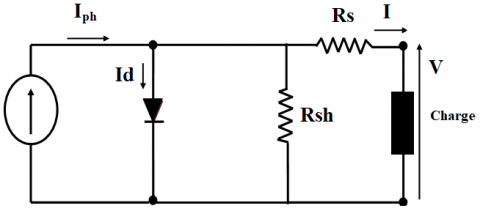Figure 2. Photovoltaic cell equivalent circuit

From the above figure, the output current is expressed in the following equation:

$i=i_{p h}-i_{s}\left[\exp \left(\frac{v+i . R_{s}}{m \frac{K T}{q}}\right)-1\right] \frac{v+i . R_{s}}{R_{s h}}$          (1)

where, ${{i}_{ph}}$ is the photo-current, is is the saturation current of the diode, m is the ideality factor, Rs and Rsh are series and parallel (shunt) resistances, T is junction temperature, K is Boltzmann constant, and q is the electron charge.

In general, for a PVG involving an array of Ns cell connected in series and Np in parallel, its output current-voltage-relation maybe deduced from the basic cell Eq. (2) addressed in [7-9] as follows:

$i=N_{p} \cdot i_{p h}-N_{p} \cdot i_{s}\left(\frac{V}{N_{s}}+\frac{R_{s} \cdot i}{N_{p}}\right)\left[\exp \left[\left(\begin{array}{c}\left.\frac{1}{m \frac{K T}{q}}\right) \\ \left(\frac{V}{N_{s}}+\frac{R_{s} \cdot i}{N_{p}}\right)\end{array}\right]-1\right]\right.$              (2)

2.2 Power generator optimization by fuzzy logic

The working principle diagram of the PV system based on fuzzy logic is shown in Figure 3 [10-12].

The control objective is to track and extract maximum power point from the PV panels for a given solar irradiation level. The maximum power corresponding to the optimum operating point is determined for different solar irradiation. Fuzzy logic controller for a PV system is adopted from widely known photovoltaic cell electrical equivalent circuit. An equivalent model for more powerful PVG made of an (Ns×Np) array of PV cells is established as in the paper .

## 3.pngFigure 3. PV system using Fuzzy logic method

2.3 Model of the centrifugal pump

The Q-H head flow characteristic of a single-stage centrifugal pump is obtained using the Pleider-Peterman model [11, 12]. The following quadratic form can approximately express the behavior of a centrifugal pump with several speeds:

$H M T=a_{0} \Omega_{r}^{2}-a_{0} \Omega_{r}-a_{0} Q^{2}$          (3)

where,

${{a}_{0}}$,${{a}_{1}}$${{a}_{2}}$ are constants data by the manufacturer.

The pump torque is given by:

$\Gamma_{r}=k_{r} \cdot \Omega_{r}^{2}$          (4)

2.4 Model of battery

Lead-acid batteries allow energy storage for several hours to a few days. The state of charge is calculated relative to the actual capacity of the nominal battery capacity, as expressed in Eq. (5) as in the paper , the general structure is given in Figure 4.

The main reaction current of the battery bank can be expressed as:

${{I}_{MR}}(t)={{I}_{bb}}(t)-{{I}_{gaz}}(t)$          (5)

where

IMR: Main battery reaction current (A);

Ibb: External battery current (A);

Igaz: Battery gassing current (A).

## 4.png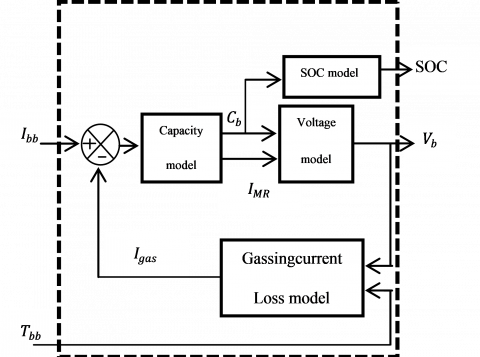Figure 4. General structure of battery model

The capacity model of the battery is less effect on the charged or the discharged current. The real battery capacity is determined as :

${{c}_{b}}(t)=\int\limits_{t=0}^{t}{{{I}_{MR}}(t)dt+}{{c}_{b}}_{,i}$           (6)

where,

Cb: Actual battery capacity (Ah);

$c_{b, i}$: Initial battery capacity (Ah).

The state-of-charge can be calculated by referring the actual capacity to the rated capacity of the battery:

$SOC=\frac{{{c}_{b}}}{{{c}_{10}}}\times 100%$       (7)

The battery voltage model is based on the “extended kinetic battery model”, which is presented by Wu et al. .

3. Modeling and Control of the Heptaphase Asynchrounous Motor

The windings of the Polyphase motor are offset by 2π / 7, generally the inductance matrices of the motor with several phases are full, which complicates the control of its last. However, like all stator or rotor inductance matrices can be diagonalized. There is therefore an orthogonal basis of eigenvectors in which the magnetic quantities of the motor are decoupled. This certainly makes it easier to control the induction motor [15-18]. Using the transformation Eq. (8) to switch from natural storage to another also makes it possible to increase the electromagnetic torque per ampere for the same volume of motor. Modeling is performed assuming linear magnetic circuits, equal mutual inductances and neglecting iron losses .

\begin{align} & {{\mathbf{T}}_{\mathbf{7}}}^{\mathbf{t}}=\left[ \begin{matrix} 1 & {{a}_{1}} & {{a}_{2}} & {{a}_{3}} & {{a}_{4}} & {{a}_{5}} & {{a}_{6}} \\ 0 & {{b}_{1}} & {{b}_{2}} & {{b}_{3}} & {{b}_{4}} & {{b}_{5}} & {{b}_{6}} \\ 1 & {{a}_{2}} & {{a}_{4}} & {{a}_{6}} & {{a}_{8}} & {{a}_{10}} & {{a}_{12}} \\ 0 & {{b}_{2}} & {{b}_{4}} & {{b}_{6}} & {{b}_{8}} & {{b}_{10}} & {{b}_{12}} \\ 1 & {{a}_{3}} & {{a}_{6}} & {{a}_{9}} & {{a}_{12}} & {{a}_{15}} & {{a}_{18}} \\ 0 & {{b}_{3}} & {{b}_{6}} & {{b}_{9}} & {{b}_{12}} & {{b}_{15}} & {{b}_{18}} \\ c & c & c & c & c & c & c \\\end{matrix} \right] \\ & with:{{a}_{h}}=\cos \left( h{2\pi }/{7}\; \right)\ ,\ {{b}_{h}}=\sin \left( h{2\pi }/{7}\; \right)\quad and\ c={1}/{\sqrt{2}}\; \\ \end{align}        (8)

The voltage equations of the seven-phase induction motor in the d-q frame are given by:

$\left\{ \begin{array}{*{35}{l}} {{v}_{ds}}={{r}_{s}}\,{{i}_{ds}}+\frac{\text{d}{{\varphi }_{ds}}}{\text{d}t}-{{\omega }_{s}}{{\varphi }_{qs}} \\ {{v}_{qs}}={{r}_{s}}\,{{i}_{qs}}+\frac{\text{d}{{\varphi }_{qs}}}{\text{d}t}+{{\omega }_{s}}{{\varphi }_{ds}} \\ {{v}_{xs}}={{r}_{s}}\,{{i}_{xs}}+\frac{\text{d}{{\varphi }_{xs}}}{\text{d}t} \\ {{v}_{ys}}={{r}_{s}}\,{{i}_{ys}}+\frac{\text{d}{{\varphi }_{ys}}}{\text{d}t} \\ {{v}_{x1s}}={{r}_{s}}\,{{i}_{x1s}}+\frac{\text{d}{{\varphi }_{x1s}}}{\text{d}t} \\ {{v}_{y1s}}={{r}_{s}}\,{{i}_{y1s}}+\frac{\text{d}{{\varphi }_{y1s}}}{\text{d}t} \\ 0={{r}_{r}}\,{{i}_{dr}}+\frac{\text{d}{{\varphi }_{dr}}}{\text{d}t}-{{\omega }_{r}}{{\varphi }_{qr}} \\ 0={{r}_{r}}\,{{i}_{qr}}+\frac{\text{d}{{\varphi }_{qr}}}{\text{d}t}+{{\omega }_{r}}{{\varphi }_{dr}} \\\end{array} \right.\quad \quad \quad \quad \quad \quad \quad$            (9)

The flux equations are given by:

$\left\{ \begin{array}{*{35}{l}} {{\varphi }_{ds}}=({{L}_{m}}+{{l}_{fs}})\,{{i}_{ds}}+{{L}_{m}}{{i}_{dr}} \\ {{\varphi }_{qs}}=({{L}_{m}}+{{l}_{fs}})\,{{i}_{qs}}+{{L}_{m}}{{i}_{qr}} \\ {{\varphi }_{xs}}={{l}_{fs}}\,{{i}_{xs}} \\ {{\varphi }_{ys}}={{l}_{fs}}\,{{i}_{ys}} \\ {{\varphi }_{x1s}}={{l}_{fs}}\,{{i}_{x1s}} \\ {{\varphi }_{y1s}}={{l}_{fs}}\,{{i}_{y1s}} \\ {{\varphi }_{dr}}=({{L}_{m}}+{{l}_{fs}})\,{{i}_{dr}}+{{L}_{m}}{{i}_{ds}} \\ {{\varphi }_{qr}}=({{L}_{m}}+{{l}_{fs}})\,{{i}_{qr}}+{{L}_{m}}{{i}_{qs}} \\\end{array} \right.$             (10)

Non-sequential components (x,y) and (x1,y1)(x1,y1) do not contribute to the torque creation, but they cannot be neglected. However, these components usually generate circulation currents when the machine is fed by an inverter. This is due to frequency harmonic of the PWM control, which do not return it to a three-phase system. A preliminary analysis made to evaluate the PWM impact on non-sequential current is show in Figure 5. The Figure 6 shows the performance of the seven phase induction machine under unbalanced parameter.

## 5a.png## 5b.pngFigure 5. Stator current of the first phase supplied with three levels inverter (a) Without non-sequential components, (b) with non-sequential components

## 6a.png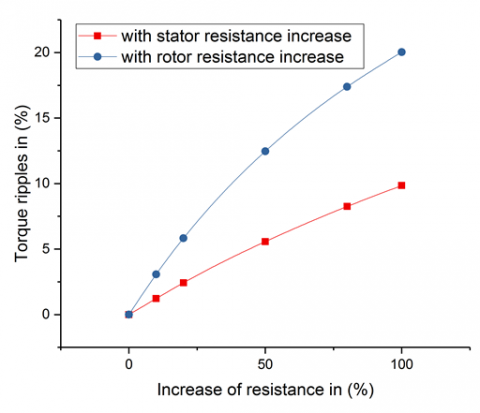## 6b.pngFigure 6. Behavior of seven phase induction machine under unbalanced parameter

The electromagnetic torque is expressed as follow:

${{\Gamma }_{em}}=p{{L}_{m}}(({{i}_{dr}}.{{i}_{qs}})-({{i}_{ds}}.{{i}_{qr}}))$           (11)

While the load torque acts as a disturbance via the following mechanical equation:

${{\Gamma }_{em}}-{{\Gamma }_{r}}=J\frac{d\Omega }{dt}$         (12)

with iqs, ids, idr, iqr, ixs, ixls, iys, iyls are respectively the stator and rotor currents components; vqs, vds, vdr, vqr, vxs, vxls, vys, vyls are the stator and rotor voltages components; $\varphi_{q s}, \varphi_{d s}, \varphi_{d r}, \varphi_{q r}, \varphi_{x s}$ $\varphi_{x l s}, \varphi_{y s}, \varphi_{y l s}$ are respectively the stator and rotor fluxes component.

3.1 Field oriented control of the seven phase induction machine

The orientation control by the rotor flux is implemented to provide the decoupling between the magnitudes of the generated electromagnetic torque and rotor flux [4, 15]. This is achieved in the rotor flux when it coincides with the d-axis of the reference with regards to the rotating field. Thus, when acting on the variables iqs, ids , the quantities Gem and jr are controlled separately. This means aligning the rotor flux vector on the d-axis: $\varphi_{d r}=\varphi_{r}$ and $\varphi_{q r}=0$.

Using this concept, the mathematical model of the seven-phase induction machine became:

$\left\{ \begin{array}{*{35}{l}} {{v}_{ds}}=\left( {{r}_{s}}+\frac{{{L}_{m}}^{2}}{{{l}_{r}}^{2}}{{r}_{r}} \right){{i}_{ds}}+\sigma {{l}_{s}}\frac{\text{d}}{\text{d}t}{{i}_{ds}}-\sigma {{l}_{s}}{{\omega }_{s}}{{i}_{qs}}-\frac{{{L}_{m}}}{{{T}_{r}}{{l}_{r}}}{{\varphi }_{r}} \\ {{v}_{qs}}=\left( {{r}_{s}}+\frac{{{L}_{m}}^{2}}{{{l}_{r}}^{2}}{{r}_{r}} \right){{i}_{qs}}+\sigma {{l}_{s}}\frac{\text{d}}{\text{d}t}{{i}_{qs}}+\sigma {{l}_{s}}{{\omega }_{s}}{{i}_{ds}}-\frac{{{L}_{m}}}{{{l}_{r}}}{{\omega }_{r}}{{\varphi }_{r}} \\ \frac{\text{d}}{\text{d}t}{{\varphi }_{r}}=\frac{{{L}_{m}}}{{{T}_{r}}}{{i}_{ds}}-\frac{1}{{{T}_{r}}}{{\varphi }_{r}} \\ {{\omega }_{s}}-{{\omega }_{r}}=\frac{{{L}_{m}}{{i}_{qs}}}{{{T}_{r}}{{\varphi }_{r}}} \\ {{\Gamma }_{em}}=p\frac{{{L}_{m}}}{{{T}_{r}}}{{\varphi }_{r}}{{i}_{qs}} \\ J\frac{\text{d}}{\text{d}t}\Omega ={{\Gamma }_{em}}-{{\Gamma }_{r}}-f\ \Omega \\\end{array} \right.$               (13)

With: J and $\Omega$ are the inertia and speed of the machine, respectively.

This induces:

\left\{\begin{aligned} \varphi_{r}^{*}=& \frac{L_{m}}{1+T_{r}} i_{d s} \\ \Gamma_{e m}^{*} &=P \frac{L_{m}}{L_{r}} i_{q s} \varphi_{r}^{*} \\ \omega_{s}^{*} &=\frac{L_{m} i_{q s}}{T_{r} \varphi_{r}^{*}+\epsilon}+\omega_{r} \end{aligned}\right.         (14)

with:

$\sigma=1-\frac{L_{m}^{2}}{L_{r} L_{s}}, T_{r}=\frac{l_{f r}+L_{m}}{r_{r}}, \epsilon=0.001$

As mentioned, the minimization of non-sequential currents is done through a controller based on fuzzy logic [19-21]. The input to these controller variables are error “E” and the derivative of error “ED”; as shown in Figure 7 and Table 1. The proposed schematics of the FOC heptaphase asynchronous motor are given in Figure 8.

## 7a.png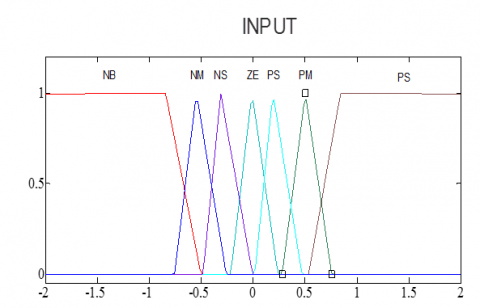(a) Input

## 7b.png(b) Output

Figure 7. Fuzzy membership of non-sequential component minimization

## 8.pngFigure 8. Improved FOC of hepataphase asynchronous motor scheme

## 9.pngFigure 9. Power of photovoltaic together with the battery power

## 10.png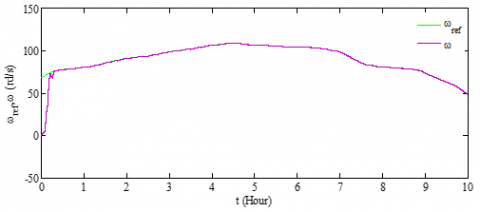Figure 10. Mechanical speed

## 11.pngFigure 11. Electromagnetic and pump torque

4. Simulation Result

The proposed photovoltaic water-pumping system is simulated in MATLAB/Simulink environment. For sizing the proposed water pumping system, an analytical method based on water needs to calculate the energy, the determination of the available solar energy and the choice of components were required. This system is elaborated to meet the needs of water for a small village in Bejaia city, situated on the southern shore of the Mediterranean, whose daily consumption is estimated to be 72 m3. Therefore, the generator consists of 32 modules in series of an energy of 60 W for each. To simulate the operation of the system, we present the radiation and temperature for a day on October whose irradiation relatively low.

Photovoltaic panel and the batteries bank are shown in the Figure 9. Figure 10 shows good performance of the direct control of the rotor flux orientation. On the other hand, the mechanical speed of the seven phase induction machine perfectly follows the reference speed derived from the power delivered by the photovoltaic panel. Figure 11 show the evolution of the seven-phase induction machine torque as well as that of the centrifugal pump. The fuzzy logic minimization of the non-sequential current components is shown in Figure 12, aided by the using of the three-level inverter. The performances of the pumping system with photovoltaic panels optimized by fuzzy logic and the batteries are shown in Figures 13-15. Figures 16 and 17 gives the climate information where the Photovoltaic panel’s irradiance of the sun are positioned and the temperature, respectively. The parameters of the seven-phase induction machine and the pumping system are given in the appendix. Table 2 gives the characteristics data of PV module, whereas, Table 3 gives the parameters of centrifugal pump.

## 12.pngFigure 12. Non-sequential currents components

## 13.pngFigure 13. Water flow and HMT

## 14.png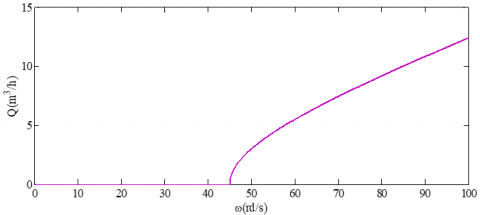Figure 14. Characteristic flow-speed

## 15.pngFigure 15. Volume of water pumped

## 16.png## 17.pngFigure 17. Temperature profile

Table 1. Rules base for fuzzy control

 dE Bn Nm sn ez sp mp bp bn Bn Bn bn bn mn sn ez nm Bn Bn bn mn sn ez sp sn Bn Bn mn sn ez sp mp ez Bn mn ns ez sp mp bp sp Mn Sn ez sp mp bp bp mp Sn Ez sp mp bp bp bp bp Ez Sp mp bp bp bp bp

Table 2. Characteristics data of PV module

 Characteristics data of PV module Power rate 60 W Voltage rate 17.1 V Current rate 3.5 A Temperature coefficients of short circuit current (0.0065±0.015) A/°C Temperature coefficients of open circuit voltage -(80±10) mV/°C

Table 3. Centrifugal pump parameters

 Parameters of the centrifugal pump Speed 157 rad/sec Pump inertia 20 g.m2 Flow 21 m3/h Height 12m a0 0.49234.10-3 m/ (rad/sec)2 a1 1,58.10-5 m(rad/sec(m2/sec)) a2 -18144 m/ (m3/sec)2
5. Conclusions

The aim of this work was the integration of the seven-phase induction machine into the water pumping system. As known, a poly-phase machine exhibits additional circulation currents (non-sequential components) when it is powered via inverters. Fuzzy logic was utilized in this paper to minimize the latter effect on the system. On the other hand, water pumping systems usually have poor energy quality which in turn justify the use of a three-level inverter. Results showed that the proposed system may give high promises in both aspects mechanical and electrical performances for water pumping systems.

Nomenclature
 S Index stator R Index rotor D Direct axe Q Quadratic axe $\mathrm{x}, \mathrm{y}, \mathrm{x}_{1}, \mathrm{y}_{1}$ No-sequential axe $\omega_{s}$ Speed of the synchronous reference frame p Number of pole pairs $\mathrm{L}_{\mathrm{m}}$ Mutual inductance $\mathrm{l}_{\mathrm{fr}}$ rotor leakage inductance $\mathrm{l}_{\mathrm{fs}}$ Stator leakage inductance J Moment of inertia $\omega_{\mathrm{r}}$ Rotor electrical angular speed Greek symbols $\Omega$ Mechanical speed $\Gamma_{\mathrm{em}}$ Electromagnetic torque $\Gamma_{\mathrm{r}}$ Load torque
Appendix

\begin{aligned} P_{n}=2.75 \mathrm{Kw} ; \mathrm{f}=& 50 \mathrm{~Hz} ; \mathrm{v}_{\mathrm{n}}=220 \mathrm{~V} ; \mathrm{i}_{\mathrm{n}}=4.8 \mathrm{~A} ; \mathrm{r}_{\mathrm{r}} =6.3 \Omega ; \mathrm{l}_{\mathrm{fs}}=0.04 \mathrm{H} ; \mathrm{l}_{\mathrm{fr}}=0.04 \mathrm{H} \end{aligned}

$\mathrm{L}_{\mathrm{m}}=0.42 \mathrm{H} ; \mathrm{J}=50 \mathrm{~g} \cdot \mathrm{m}^{2} ; \mathrm{k}_{\mathrm{f}}=1.210^{-3} \mathrm{Nm} . \mathrm{s} / \mathrm{rd}$

References

 Campana, P.E., Li, H., Zhang, J., Zhang, R., Liu, J., Yan, J. (2015). Economic optimization of photovoltaic water pumping systems for irrigation. Energy Conversion and Management, 95: 32-41. https://doi.org/10.1016/j.enconman.2015.01.066

 Abdel-Khalik, A.S., Masoud, M.I., Williams, B.W. (2012). Vector controlled multiphase induction machine: Harmonic injection using optimized constant gains. Electric Power Systems Research, 89: 116-128. https://doi.org/10.1016/j.epsr.2012.03.001

 Rizwan Khan, M., Iqbal, A., Ahmad, M. (2008). MRAS-based sensorless control of a vector controlled five-phase induction motor drive. Electric Power Systems Research, 78(8): 1311-1321. https://doi.org/10.1016/j.epsr.2007.11.006

 Singh, G.K. (2002). Multi-phase induction machine drive research- a survey. Electric Power Systems Research, 61(2): 139-147. https://doi.org/10.1016/S0378-7796(02)00007-X

 Kong, W., Huang, J., Kang, M., Li, B., Zhao, L. (2014). Fault-tolerant control of five-phase induction motor under single-phase open. Journal of Electrical Engineering and Technology, 9(3): 742-750. https://doi.org/10.5370/JEET.2014.9.3.899

 Betin, F., Capolino, G.A. (2008). Modeling and control of six-phase symmetrical induction machine under fault condition due to open phases. IEEE Transactions on Industrial Electronics, 55(5): 1966-1977. https://doi.org/10.1109/TIE.2008.918479

 Kumar, N., Saha, T.K., Dey, J. (2016). Modeling control and analysis of cascaded inverter based grid-connected photovoltaic system. Electrical Power and Energy Systems, 78: 165-173. https://doi.org/10.1016/j.ijepes.2015.11.092

 Rahrah, K., Rekioua, D., Rekioua, T., Bacha, S. (2015). Photovoltaic pumping system in Bejaia climate with battery storage. International Journal of Hydrogen Energy, 40(39): 13665-13675. https://doi.org/10.1016/j.ijhydene.2015.04.048

 Singh, B., Sharma, U., Kumar, S. (2018). Standalone photovoltaic water pumping system using induction motor drive with reduced sensor. IEEE Transaction on industrie Application, 54(4): 3645-3655. https://doi.org/10.1109/TIA.2018.2825285

 Camocardi, P., Battaiotto, P., Mantz, R. (2010). Autonomous BDFIG-wind generator with torque and pitch control for maximum efficiency in a water pumping system. International Journal of Hydrogen Energy, 35(11): 5778-5785. https://doi.org/10.1016/j.ijhydene.2010.02.099

 Iffouzar, K., Taraft, S., Aouzellag, H., Ghedamsi, K., Aouzellag, D. (2015). Behavior of a six phase induction motor fed by multilevel inverter. 4th International Conference on Electrical Engineering (ICEE), Boumerdes, pp. 1-7. https://doi.org/10.1109/INTEE.2015.7416779

 Gonzalez, I., Ramiro, A., Calderon, M., Calderon, A.J., Gonzalez, J.F. (2012). Estimation of the state-of-charge of gel lead-acid batteries and application to the control of a stand-alone wind-solar test-bed with hydrogen support. International Journal of Hydrogen Energy, 37(15): 11090-11103. https://doi.org/10.1016/j.ijhydene.2012.05.001

 Esram, T., Chapman, P.L. (2007). Comparison of photovoltaic array maximum power point tracking techniques. IEEE Trans. Energy Conv., 22(2): 439-449. https://doi.org/10.1109/TEC.2006.874230

 Wu, J.C., Wu, K.D., Jou, H.L.,Wu, Z.H., Chang, S.K. (2013). Novel power electronic interface for grid-connected fuel cell power generation system. Energy Conversion and Management, 71: 227-234. https://doi.org/10.1016/j.enconman.2013.03.038

 Iffouzar, K., Taraft, S., Aouzellag, H., Aouzellag, D., Ghedamsi, K. (2015). Improved DRFOC of polyphase induction motor based on fuzzy logic controller speed. 4th International Conference on Electrical Engineering (ICEE), Boumerdes, Algeria. https://doi.org/10.1109/INTEE.2015.7416780

 Levi, E., Bojoi, R., Profumo, F., Toliyat, H.A., Williamson, S. (2007). Multiphase induction motor drives - a technology status review. IET Electric Power Applications, 1(4): 489. https://doi.org/10.1049/iet-epa:20060342

 Khaldi, L., Iffouzar, K., Ghedamsi, K., Aouzellag, D. (2019). Performance analysis of five-phase induction machine under unbalanced parameters. Journal Européen des Systèmes Automatisés, 52(5): 521-526. https://doi.org/10.18280/jesa.520512

 Xu, H., Toliyat, H., Petersen, L.J. (2002). Five-phase induction motor drives with DSP-based control system. IEEE Transactions on Power Electronics, 17(4): 524-533. https://doi.org/10.1109/TPEL.2002.800983

 Tabanjat, A., Becherif, M., Emziane, M., Hissel, D., Ramadan, H.S., Mahmah, B. (2015). Fuzzy logic-based water heating control methodology for the efficiency enhancement of hybrid PV-PEM electrolyser systems. International Journal of Hydrogen Energy, 40(5): 2149-2161. https://doi.org/10.1016/j.ijhydene.2014.11.135

 Eren, Y., Erdinc, O., Gorgun, H., Uzunoglu, M., Vural, B.A. (2009). Fuzzy logic based supervisory controller for an FC/UC hybrid vehicular power system. International Journal of Hydrogen Energy, 34(20): 8681-8694. https://doi.org/10.1016/j.ijhydene.2009.08.033

 Lekhchine, S., Bahi, S., Souﬁ, Y. (2014). Indirect rotor ﬁeld oriented control based on fuzzy logic controlled double star induction machine. Electrical Power and Energy Systems, 57: 206-211. https://doi.org/10.1016/j.ijepes.2013.11.053